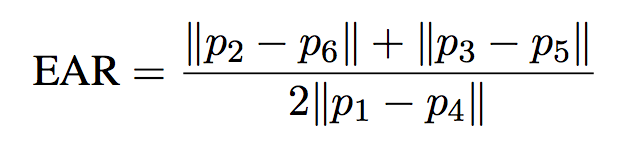Trusted answers to developer questions
Trusted Answers to Developer Questions

Related Tags

python
opencv
dlib
machine learning
communitycreator

# Eye Blink detection using OpenCV, Python, and Dlib

Grokking Modern System Design Interview for Engineers & Managers

Ace your System Design Interview and take your career to the next level. Learn to handle the design of applications like Netflix, Quora, Facebook, Uber, and many more in a 45-min interview. Learn the RESHADED framework for architecting web-scale applications by determining requirements, constraints, and assumptions before diving into a step-by-step design process.Let’s start working on the code in chunks:## 1. Import required libraries

import argparse
import time

import cv2
import dlib
import imutils
import numpy as np
from imutils import face_utils
from imutils.video import FileVideoStream, VideoStream
from scipy.spatial import distance as dist

• imutils library : FileVideoStream, VideoStream

• dlib : Contains an implementation of facial landmark detection

• cv2 : OpenCV library

## 2. Function to calculate EAR as per the research paperdef eye_aspect_ratio(eye):
A = dist.euclidean(eye, eye)
B = dist.euclidean(eye, eye)
C = dist.euclidean(eye, eye)
ear = (A + B) / (2.0 * C)
return ear


## 3. Passing command-line arguments

ap = argparse.ArgumentParser()
"-p", "--shape-predictor", required=True, help="path to facial landmark predictor"
)
ap.add_argument("-v", "--video", type=str, default="", help="path to input video file")
args = vars(ap.parse_args())

• shape-predictor: This is the path to dlib's pre-trained facial landmark detector.

• video: Optional switch controls the path to an input video file residing on disk.

### 4. Important variables to tune the implementation

EYE_AR_THRESH = 0.3
EYE_AR_CONSEC_FRAMES = 3

COUNTER = 0
TOTAL = 0

• EYE_AR_THRESH: If the eye aspect ratio falls below a certain threshold and then rises above the threshold, we’ll register a “blink”

• EYE_AR_CONSEC_FRAME: This value is set to $3$ to indicate that three successive frames with an eye aspect ratio less than EYE_AR_THRESH must happen in order for a blink to be registered.

• COUNTER: The total number of successive frames that have an eye aspect ratio less than EYE_AR_THRESH.

• TOTAL: The number of blinks that have taken place while the script is running.

### 5. Initialize dlib’s face detector and facial landmark detector

print("[INFO] loading facial landmark predictor...")
detector = dlib.get_frontal_face_detector()
predictor = dlib.shape_predictor(args["shape_predictor"])

(lStart, lEnd) = face_utils.FACIAL_LANDMARKS_IDXS["left_eye"]
(rStart, rEnd) = face_utils.FACIAL_LANDMARKS_IDXS["right_eye"]


### 6. Start the video streaming thread

print("[INFO] starting video stream thread...")
vs = FileVideoStream(args["video"]).start()
fileStream = True
vs = VideoStream(src=0).start()
fileStream = False
time.sleep(1.0)


FileVideoStream for disk videos and VideoStream for video from the webcam.

### 7. Main loop

while True:

if fileStream and not vs.more():
break

frame = imutils.resize(frame, width=800)
gray = cv2.cvtColor(frame, cv2.COLOR_BGR2GRAY)

rects = detector(gray, 0)


We start looping over frames from our video stream. If we are accessing a video file stream and there are no more frames left in the video, we break from the loop.

Line 61 reads the frame by resizing it and converting it to grayscale (Lines 62 and 63), then it detects faces in the grayscale frame on Line 65 via dlib’s built-in face detector.

### 8. Loop over each of the faces in the frame and then apply facial landmark detection to each of them

   for rect in rects:

shape = predictor(gray, rect)
shape = face_utils.shape_to_np(shape)

leftEye = shape[lStart:lEnd]
rightEye = shape[rStart:rEnd]
leftEAR = eye_aspect_ratio(leftEye)
rightEAR = eye_aspect_ratio(rightEye)

ear = (leftEAR + rightEAR) / 2.0


### 9. Visualizing the facial landmarks for the eye regions themselves

       leftEyeHull = cv2.convexHull(leftEye)
rightEyeHull = cv2.convexHull(rightEye)
cv2.drawContours(frame, [leftEyeHull], -1, (0, 255, 0), 1)
cv2.drawContours(frame, [rightEyeHull], -1, (0, 255, 0), 1)



### 10. Counting Blinks

        if ear < EYE_AR_THRESH:
COUNTER += 1

else:

if COUNTER >= EYE_AR_CONSEC_FRAMES:
TOTAL += 1

COUNTER = 0


### 11. Drawing Blinks and EAR on the stream

cv2.putText(
frame,
(10, 30),
cv2.FONT_HERSHEY_SIMPLEX,
0.7,
(0, 0, 255),
2,
)
cv2.putText(
frame,
"EAR: {:.2f}".format(ear),
(300, 30),
cv2.FONT_HERSHEY_SIMPLEX,
0.7,
(0, 0, 255),
2,
)


### Complete code

import argparseimport timeimport cv2import dlibimport imutilsimport numpy as npfrom imutils import face_utilsfrom imutils.video import FileVideoStream, VideoStreamfrom scipy.spatial import distance as distdef eye_aspect_ratio(eye):    A = dist.euclidean(eye, eye)    B = dist.euclidean(eye, eye)    C = dist.euclidean(eye, eye)    ear = (A + B) / (2.0 * C)    return earap = argparse.ArgumentParser()ap.add_argument(    "-p", "--shape-predictor", required=True, help="path to facial landmark predictor")ap.add_argument("-v", "--video", type=str, default="", help="path to input video file")args = vars(ap.parse_args())EYE_AR_THRESH = 0.3EYE_AR_CONSEC_FRAMES = 3COUNTER = 0TOTAL = 0print("[INFO] loading facial landmark predictor...")detector = dlib.get_frontal_face_detector()predictor = dlib.shape_predictor(args["shape_predictor"])(lStart, lEnd) = face_utils.FACIAL_LANDMARKS_IDXS["left_eye"](rStart, rEnd) = face_utils.FACIAL_LANDMARKS_IDXS["right_eye"]print("[INFO] starting video stream thread...")vs = FileVideoStream(args["video"]).start()fileStream = Truevs = VideoStream(src=0).start()fileStream = Falsetime.sleep(1.0)while True:    if fileStream and not vs.more():        break    frame = vs.read()    frame = imutils.resize(frame, width=800)    gray = cv2.cvtColor(frame, cv2.COLOR_BGR2GRAY)    rects = detector(gray, 0)    for rect in rects:        shape = predictor(gray, rect)        shape = face_utils.shape_to_np(shape)        leftEye = shape[lStart:lEnd]        rightEye = shape[rStart:rEnd]        leftEAR = eye_aspect_ratio(leftEye)        rightEAR = eye_aspect_ratio(rightEye)        ear = (leftEAR + rightEAR) / 2.0        leftEyeHull = cv2.convexHull(leftEye)        rightEyeHull = cv2.convexHull(rightEye)        cv2.drawContours(frame, [leftEyeHull], -1, (0, 255, 0), 1)        cv2.drawContours(frame, [rightEyeHull], -1, (0, 255, 0), 1)        if ear < EYE_AR_THRESH:            COUNTER += 1        else:            if COUNTER >= EYE_AR_CONSEC_FRAMES:                TOTAL += 1            COUNTER = 0        cv2.putText(            frame,            "Blinks: {}".format(TOTAL),            (10, 30),            cv2.FONT_HERSHEY_SIMPLEX,            0.7,            (0, 0, 255),            2,        )        cv2.putText(            frame,            "EAR: {:.2f}".format(ear),            (300, 30),            cv2.FONT_HERSHEY_SIMPLEX,            0.7,            (0, 0, 255),            2,        )    cv2.imshow("Frame", frame)    key = cv2.waitKey(1) & 0xFF    if key == ord("q"):        breakcv2.destroyAllWindows()vs.stop()

You can run the above code using the following commands:

• For live video:
python3 detect_blinks.py --shape-predictor shape_predictor_68_face_landmarks.dat

• For demo video:
python3 detect_blinks.py --shape-predictor shape_predictor_68_face_landmarks.dat --video “replace this with demo video file path”


The complete code can also be found here.

RELATED TAGS

python
opencv
dlib
machine learning
communitycreator

CONTRIBUTOR